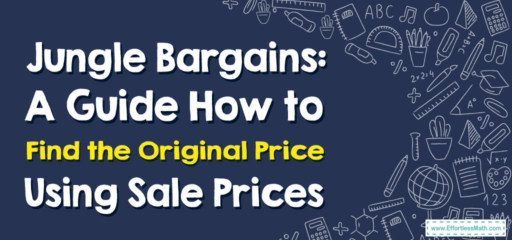# Jungle Bargains: A Guide How to Find the Original Price Using Sale Prices

Hello, intrepid jungle explorers!

On our expedition today, we're not just searching for ancient artifacts or rare species, but we're also on a hunt for jungle bargains! Finding great deals is fun, but do you know how to figure out the original price from the sale price? Let's dive into the underbrush and learn how!## 1. Welcome to the Jungle: Understanding Original and Sale Prices

Before we start our hunt, let’s learn the lingo:

• Original Price: This is the initial price of an item before any discounts are applied.
• Sale Price: This is the price of an item after discounts are applied.

## 2. Jungle Hunt: Finding the Original Price Using Sale Prices

Now, with our compasses and maps ready, let’s start our jungle bargain hunt!

### Expedition Guide: Finding the Original Price Using Sale Prices

#### Step 1: Identify the Sale Price

First, identify the sale price of the item. This is the amount you’ll pay after the discount.

#### Step 2: Understand the Discount Percentage

Figure out the discount rate that was applied to the item. This is usually provided as a percentage.

#### Step 3: Calculate the Original Price

Finally, calculate the original price using the sale price and the discount rate.

For instance, if you buy a parrot for $$45$$ at a $$10$$$$\%$$ off discount, what was the original price?

1. Identify the Sale Price: The sale price of the parrot is $$45$$.
2. Understand the Discount Percentage: The discount rate is $$10$$$$\%$$, or $$0.10$$ as a decimal.
3. Calculate the Original Price: Since the sale price is $$90$$$$\%$$ of the original price ($$100$$$$\%$$$$-\ 10$$$$\%$$), we can find the original price by dividing the sale price by $$90$$$$\%$$ (or $$0.90$$ as a decimal). So, $$\frac{45}{0.90} = 50$$.

And just like that, we’ve successfully navigated the jungle of sale prices! Remember, fellow explorers, not all treasures in the jungle are gold or gems – sometimes, they’re fantastic bargains! Until our next jungle expedition, happy hunting!

### What people say about "Jungle Bargains: A Guide How to Find the Original Price Using Sale Prices - Effortless Math: We Help Students Learn to LOVE Mathematics"?

No one replied yet.

X
30% OFF

Limited time only!

Save Over 30%

SAVE $5 It was$16.99 now it is \$11.99